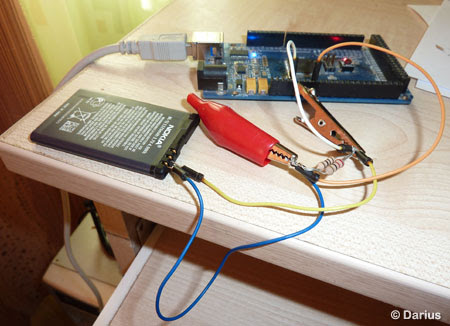# Circuit Lake

Electronic Project and Circuit Collection

## Arduino Lithium-ion Battery Capacity Tester

05/08/2012 Category: Arduino, Measurement, Microcontroller, Monitoring, Project

This project shows you how to build a simple Li-on Battery Capacity Tester. It consists of single resistor that discharges battery. Arduino measures the voltage drop across resistor. According to Ohm’s Law current = voltage/resistance. Every second value of current is divided by 3600 and summed up to get the capacity expressed in Ah (Amp per hour). Discharging progress then transmitted via serial port and user can see the result through Bray’s Terminal.

This battery tester used two parallel connected resistors that total resistance is 6.9 ohm. He warns to use proper power rating to avoid burned resistor. If voltage across 6.9 ohm resistor is 3.7 V, then current = 0.54 A, so use resistor with power rating > 2W.Darius, project designer, said that Li-ion battery voltage will drop constantly to about ~ 2V since battery integrated circuit will cuts off at this value to prevent cell from over discharging. A reference voltage of ADC of Arduino is 5V, so make sure that batteries voltage is bellow that value, or use voltage divider.

Battery Capacity Meter
Project Firmware, Schematic and Documentation
electronicsblog.net